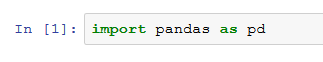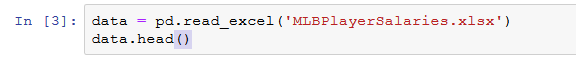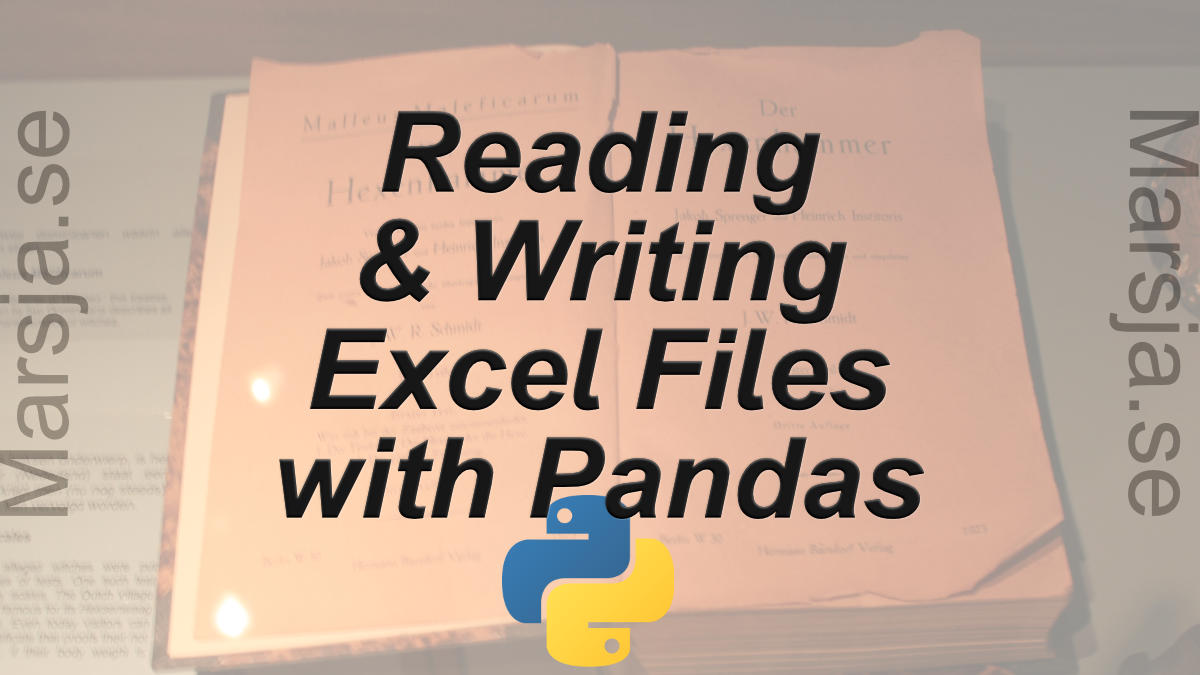52 Shares

In this Pandas tutorial, we will learn how to work with Excel files (e.g., xls) in Python. It will provide an overview of how to use Pandas to load xlsx files and write spreadsheets to Excel.

In the first section, we will go through, with examples, how to use Pandas read_excel to; 1) read an Excel file, 2) read specific columns from a spreadsheet, 3) read multiple spreadsheets, and combine them to one dataframe. Furthermore, we are going to learn how to read many Excel files, and how to convert data according to specific data types (e.g., using Pandas dtypes).

When we have done this, we will continue by learning how to use Pandas to write Excel files; how to name the sheets and how to write to multiple sheets. Make sure to check out the newer post about reading xlsx files in Python with openpyxl, as well.

## How to Install Pandas

Before we continue with this Pandas read and write Excel files tutorial there is something we need to do; installing Pandas (and Python, of course, if it’s not installed). We can install Pandas using Pip, given that we have Pip installed, that is. See here how to install pip.

```.wp-block-code {
border: 0;
}

.wp-block-code > div {
overflow: auto;
}

.shcb-language {
border: 0;
clip: rect(1px, 1px, 1px, 1px);
-webkit-clip-path: inset(50%);
clip-path: inset(50%);
height: 1px;
margin: -1px;
overflow: hidden;
position: absolute;
width: 1px;
word-wrap: normal;
word-break: normal;
}

.hljs {
box-sizing: border-box;
}

.hljs.shcb-code-table {
display: table;
width: 100%;
}

.hljs.shcb-code-table > .shcb-loc {
color: inherit;
display: table-row;
width: 100%;
}

.hljs.shcb-code-table .shcb-loc > span {
display: table-cell;
}

.wp-block-code code.hljs:not(.shcb-wrap-lines) {
white-space: pre;
}

.wp-block-code code.hljs.shcb-wrap-lines {
white-space: pre-wrap;
}

.hljs.shcb-line-numbers {
border-spacing: 0;
counter-reset: line;
}

.hljs.shcb-line-numbers > .shcb-loc {
counter-increment: line;
}

.hljs.shcb-line-numbers .shcb-loc > span {
}

.hljs.shcb-line-numbers .shcb-loc::before {
border-right: 1px solid #ddd;
content: counter(line);
display: table-cell;
text-align: right;
-webkit-user-select: none;
-moz-user-select: none;
-ms-user-select: none;
user-select: none;
white-space: nowrap;
width: 1%;
}
```# Linux Users
pip install pandas

# Windows Users
python pip install pandas```Code language: Bash (bash)```

Note, if pip is telling us that there’s a newer version of pip, we may want to upgrade it. In a recent post, we cover how to upgrade pip to the latest version. Finally, before going on to the next section, you can use pip to install a certain version (i.e., older) of a packages usch as Pandas.

### Installing Anaconda Scientific Python Distribution

Another great option is to consider is to install the Anaconda Python distribution. This is really an easy and fast way to get started with computer science. No need to worry about installing the packages you need to do computer science separately.

Both of the above methods are explained in this tutorial. Now, in a more recent blog post, we also cover how to install a Python package using pip, conda, and Anaconda. In that post, you will find more information about installing Python packages.

## How to Read Excel Files to Pandas Dataframes:

Can Pandas read xlsx files? The short answer is, of course, “yes”. In this section, we are going to learn how to read Excel files and spreadsheets to Pandas dataframe objects. All examples in this Pandas Excel tutorial use local files. Note, that read_excel also can also load Excel files from a URL to a dataframe.  As always when working with Pandas, we have to start by importing the module:

``import pandas as pd`Code language: Python (python)`

Now it’s time to learn how to use Pandas read_excel to read in data from an Excel file. The easiest way to use this method is to pass the file name as a string. If we don’t pass any other parameters, such as sheet name, it will read the first sheet in the index. In the first example, we are not going to use any parameters:

``````# Pandas read xlsx
df.head()```Code language: Python (python)```

Here, Pandas read_excel method read the data from the Excel file into a Pandas dataframe object. We then stored this dataframe into a variable called df.

When using read_excel Pandas will, by default, assign a numeric index or row label to the dataframe, and as usual, when int comes to Python, the index will start with zero. We may have a reason to leave the default index as it is.

For instance, if your data doesn’t have a column with unique values that can serve as a better index. In case there is a column that would serve as a better index, we can override the default behavior.

### Setting the Index Column when Reading xls File

This is done by setting the index_col parameter to a column. It takes a numeric value for setting a single column as index or a list of numeric values for creating a multi-index. In the example below, we use the column ‘Player’ as indices. Note, these are not unique and it may, thus, not make sense to use these values as indices.

``df = pd.read_excel('MLBPlayerSalaries.xlsx', sheet_names='MLBPlayerSalaries', index_col='Player')`Code language: Python (python)`

Now, if one or two of your columns, for instance, are objects you use Pandas to_datetime to convert a column, properly.

### Importing an Excel File to Pandas in Two Easy Steps:

Time needed: 1 minute.

Here’s a quick answer to the How do you import an Excel file into Python using Pandas? Importing an Excel file into a Pandas dataframe basically only requires two steps, given that we know the path, or URL, to the Excel file:

1. Import Pandas

In the script type import pandas as pd2. Use Pandas read_excel method

Next step is to type df = pd.read_excel(FILE_PATH_OR_URL)
Remember to change FILE_PATH_OR_URL to the path or the URL of the Excel file.Now that we know how easy it is to load an Excel file into a Pandas dataframe we are going to continue with learning more about the read_excel method.

### Reading Specific Columns using Pandas read_excel

When using Pandas read_excel we will automatically get all columns from an Excel file. If we, for some reason, don’t want to parse all columns in the Excel file, we can use the parameter usecols. Let’s say we want to create a dataframe with the columns PlayerSalary, and Position, only. We can do this by adding 1, 3, and 4 in a list:

``````cols = [1, 2, 3]

df = pd.read_excel('MLBPlayerSalaries.xlsx', sheet_names='MLBPlayerSalaries', usecols=cols)
df.head()```Code language: Python (python)```

According to the read_excel documentation, we should be able to put in a string. For instance, cols=’Player:Position’ should give us the same results as above.

### Handling Missing Data using Pandas read_excel

If our data has missing values in some cells and these missing values are coded in some way, like “Missing” we can use the na_values parameter.

#### Pandas Read Excel Example with Missing Data

In the example below, we are using the parameter na_values and we are putting in a string (i.e., “Missing’):

``````df = pd.read_excel('MLBPlayerSalaries_MD.xlsx', na_values="Missing", sheet_names='MLBPlayerSalaries', usecols=cols)
df.head()```Code language: Python (python)```

In the read excel examples above we used a dataset that can be downloaded from this page.

### How to Skip Rows when Reading an Excel File

Now we will learn how to skip rows when loading an Excel file using Pandas. For this read excel example, we will use data that can be downloaded here.

In the following Pandas read_excel example we load the sheet ‘session1’, which contains rows that we need to skip (these rows contain some information about the dataset).

We will use the parameter sheet_name=’Session1′ to read the sheet named ‘Session1’ (the example data contains more sheets; e.g., ‘Session2’ will load that sheet). Note, the first sheet will be read if we don’t use the sheet_name parameter. In this example, the important part is the parameter skiprow=2. We use this to skip the first two rows:

``````df = pd.read_excel('example_sheets1.xlsx', sheet_name='Session1', skiprows=2)
df.head()```Code language: Python (python)```

Another way to get Pandas read_excel to read from the Nth row is by using the header parameter. In the example Excel file, we use here, the third row contains the headers and we will use the parameter header=2 to tell Pandas read_excel that our headers are on the third row.

``df = pd.read_excel('example_sheets1.xlsx', sheet_name='Session1', header=2)`Code language: Python (python)`

Now, if we want Pandas read_excel to read from the second row, we change the number in the skiprows and header arguments to 2, and so on.

## Reading Multiple Excel Sheets to Pandas Dataframes

In this section, of the Pandas read excel tutorial, we are going to learn how to read multiple sheets. Our Excel file, example_sheets1.xlsx’, has two sheets: ‘Session1’, and ‘Session2.’ Each sheet has data from an imagined experimental session. In the next example we are going to read both sheets, ‘Session1’ and ‘Session2’. Here’s how to use Pandas read_excel to read multiple sheets:

``````df = pd.read_excel('example_sheets1.xlsx',
sheet_name=['Session1', 'Session2'], skiprows=2)```Code language: Python (python)```

By using the parameter sheet_name, and a list of names, we will get an ordered dictionary containing two dataframes:

``df`Code language: Python (python)`

When working with Pandas read_excel we may want to join the data from all sheets (in this case sessions). Merging Pandas dataframes are quite easy; we just use the concat function and loop over the keys (i.e., sheets):

``df2 = pd.concat(df[frame] for frame in data.keys())`Code language: Python (python)`

Now in the example Excel file, there is a column identifying the dataset (e.g., session number). However, maybe we don’t have that kind of information in our Excel file. To merge the two dataframes and adding a column depicting which session we can use a for loop:

``````dfs = []
for framename in data.keys():
temp_df = data[framename]
temp_df['Session'] = framename
dfs.append(temp_df)

df = pd.concat(dfs)```Code language: Python (python)```

In the code above, we start by creating a list and continue by looping through the keys in the list of dataframes. Finally, we create a temporary dataframe and take the sheet name and add it in the column ‘Session’.

### Pandas Read Excel: How to Read All Sheets

Now, it is, of course, possible that when we want to read multiple sheets we also want to read all the sheets in the Excel file. That is, if we want to use read_excel to load all sheets from an Excel file to a dataframe it is possible. When reading multiple sheets and we want all sheets we can set the parameter sheet_name to None.

``all_sheets_df = pd.read_excel('example_sheets1.xlsx', sheet_name=None)`Code language: Python (python)`

# Pandas Read Excel: Reading Many Excel Files

In this section, of the Pandas read excel tutorial, we will learn how to load many files into a Pandas dataframe because, in some cases, we may have a lot of Excel files containing data from, let’s say, different experiments. In Python, we can use the modules os and fnmatch to read all files in a directory. Finally, we use list comprehension to use read_excel on all files we found:

``````import os, fnmatch
xlsx_files = fnmatch.filter(os.listdir('.'), '*concat*.xlsx')

dfs = [pd.read_excel(xlsx_file) for xlsx_file in xlsx_files]```Code language: Python (python)```

If it makes sense we can, again, use the function concat to merge the dataframes:

``df = pd.concat(dfs, sort=False)`Code language: Python (python)`

There are other methods for reading many Excel files and merging them. We can, for instance, use the module glob together with Pandas concat to read multiple xlsx files:

``````import glob
list_of_xlsx = glob.glob('./*concat*.xlsx')
df = pd.concat(list_of_xlsx)```Code language: Python (python)```

Note, the files in this example, where we read multiple xlsx files using Pandas, are located here. They are named example_concat.xlsx, example_concat1.xlsx, and example_concat3.xlsx and should be added to the same directory as the Python script. Another option, of course, is to add the file path to the files. E.g., if we want to read multiple Excel files, using Pandas read_excel method, and they are stored in a directory called “SimData” we would do as follows:

``````import glob
list_of_xlsx = glob.glob('./SimData/*concat*.xlsx')
df = pd.concat(list_of_xlsx)```Code language: Python (python)```

## Setting the Data Type for Data or Columns

If we need to, we can also, set the data type for the columns when reading Excel files using Pandas. Let’s use Pandas to read the example_sheets1.xlsx again. In the Pandas read_excel example below we use the dtype parameter to set the data type of some of the columns.

``````df = pd.read_excel('example_sheets1.xlsx',sheet_name='Session1',
'Mean':int, 'Session':str})```Code language: Python (python)```

We can use the method info to see the what data types the different columns have:

``df.info()`Code language: Python (python)`

## Writing Pandas Dataframes to Excel

Excel files can, of course, be created in Python using Pandas to_excel method. In this section of the post, we will learn how to create an excel file using Pandas. First, before writing an Excel file, we will create a dataframe containing some variables. Before that, we need to import Pandas:

``import pandas as pd`Code language: Python (python)`

The next step is to create the dataframe. We will create the dataframe using a dictionary. The keys will be the column names and the values will be lists containing our data:

``````df = pd.DataFrame({'Names':['Andreas', 'George', 'Steve',
'Sarah', 'Joanna', 'Hanna'],
'Age':[21, 22, 20, 19, 18, 23]})```Code language: Python (python)```

In this Pandas write to Excel example, we will write the dataframe to an Excel file using the to_excel method. Noteworthy, when using Pandas to_excel in the code chunk below, we don’t use any parameters.

``df.to_excel('NamesAndAges.xlsx')`Code language: Python (python)`

In the Excel file created when using Pandas to_excel is shown below. Evidently, if we don’t use the parameter sheet_name we get the default sheet name, ‘Sheet1’. Now, we can also see that we get a new column in our Excel file containing numbers. These are the index from the dataframe.

If we want our sheet to be named something else and we don’t want the index column we can add the following argument and parameters when we use Pandas to write to Excel:

``df.to_excel('NamesAndAges.xlsx', sheet_name='Names and Ages', index=False)`Code language: Python (python)`

### Writing Multiple Pandas Dataframes to an Excel File:

In this section, we are going to use Pandas ExcelWriter and Pandas to_excel to write multiple Pandas dataframes to one Excel file. That is if we happen to have many dataframes that we want to store in one Excel file but on different sheets, we can do this easily. However, we need to use Pandas ExcelWriter now:

``````df1 = pd.DataFrame({'Names': ['Andreas', 'George', 'Steve',
'Sarah', 'Joanna', 'Hanna'],
'Age':[21, 22, 20, 19, 18, 23]})

df2 = pd.DataFrame({'Names': ['Pete', 'Jordan', 'Gustaf',
'Sophie', 'Sally', 'Simone'],
'Age':[22, 21, 19, 19, 29, 21]})

df3 = pd.DataFrame({'Names': ['Ulrich', 'Donald', 'Jon',
'Jessica', 'Elisabeth', 'Diana'],
'Age':[21, 21, 20, 19, 19, 22]})

dfs = {'Group1':df1, 'Group2':df2, 'Group3':df3}
writer = pd.ExcelWriter('NamesAndAges.xlsx', engine='xlsxwriter')

for sheet_name in dfs.keys():
dfs[sheet_name].to_excel(writer, sheet_name=sheet_name, index=False)

writer.save()```Code language: Python (python)```

In the code above, we create 3 dataframes and then we continue to put them in a dictionary. Note, the keys are the sheet names and the cell names are the dataframes. After this is done we create a writer object using the xlsxwriter engine. We then continue by looping through the keys (i.e., sheet names) and add each sheet. Finally, the file is saved. Note, the final step is important as leaving this out will not give you the intended results.

Of course, there are other ways to store data. One of them is using JSON files. See the latest tutorial on how to read and write JSON files using Pandas to learn about one way to load and save data in the JSON format.

More resources on how to load data in different formats:

## Summary: How to Work With Excel Files using Pandas

That was it! In this post, we have learned a lot! We have, among other things, learned how to:

• Load Excel files to dataframes:
• Read Excel sheets and skip rows
• Merging many sheets to a dataframe
• Loading many Excel files into one dataframe
• Write a dataframe to an Excel file
• Taking many dataframes and writing them to one Excel file with many sheets

Leave a comment below if you have any requests or suggestions on what should be covered next! Check the post A Basic Pandas Dataframe Tutorial for Beginners to learn more about working with Pandas dataframe. That is after you have loaded them from a file (e.g., Excel spreadsheets)52 Shares